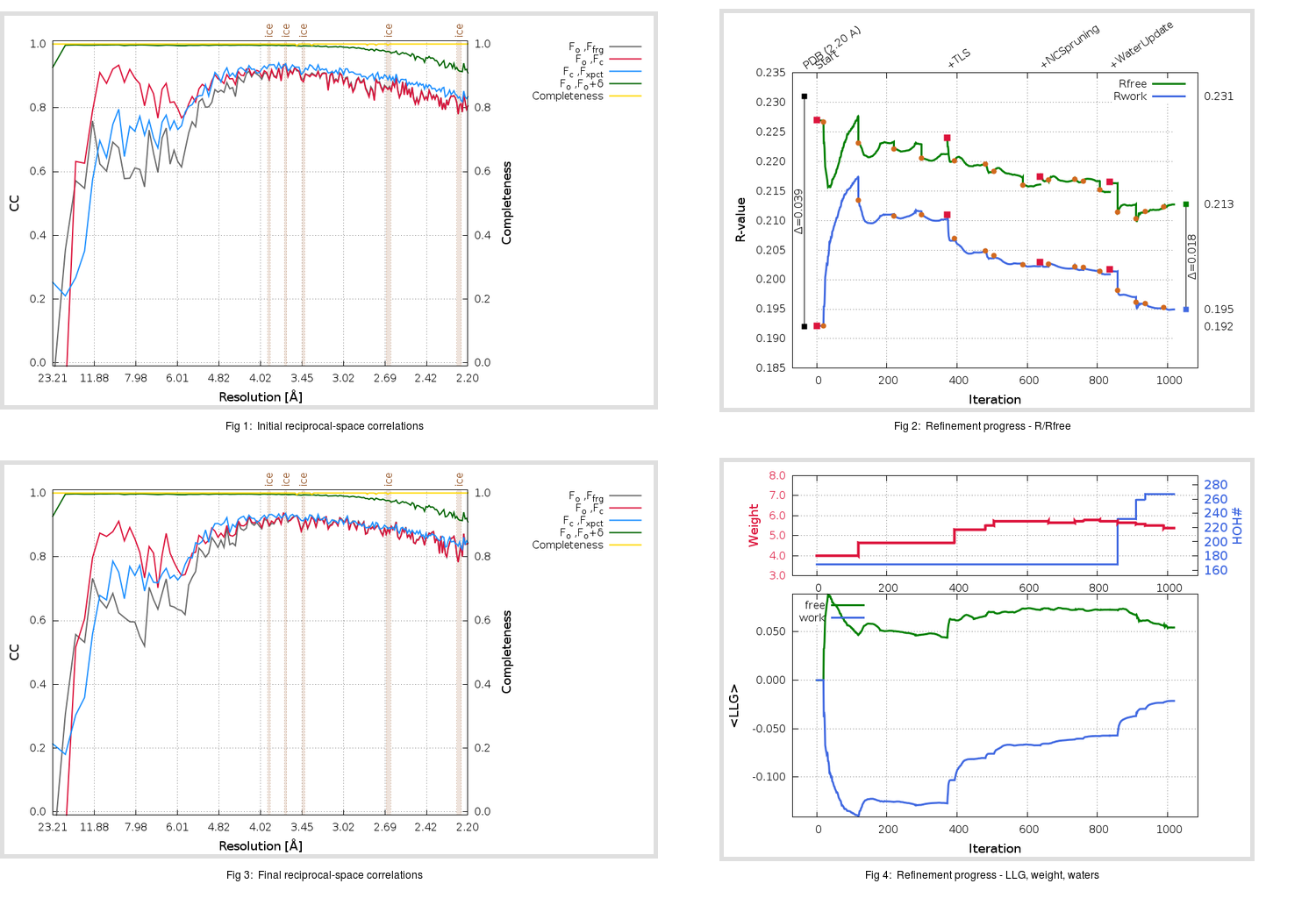Content:

```    Diffraction limits & principal axes of ellipsoid fitted to diffraction cut-off surface:
2.142         0.9993   0.0000  -0.0377       0.949 a* - 0.315 c*
2.165         0.0000   1.0000   0.0000       b*
2.159         0.0377   0.0000   0.9993       0.030 a* + c*
```

## Deposited

` `
 Date deposited Date data collection Resolution R, Rfree 20111231 20090916 2.20 0.1890 0.2310

Molprobity (CCP4 7.0 version) summary:

```Ramachandran outliers =   0.00 %
favored =  95.69 %
Rotamer outliers      =   1.92 %
C-beta deviations     =     0
Clashscore            =   5.43
RMS(bonds)            =   0.0054
RMS(angles)           =   0.94
MolProbity score      =   1.81
Resolution            =   2.20
R-work                =   0.1890
R-free                =   0.2310
```

```Number of waters      =   168

<B> (all atoms) =   34.38 ( sd =   11.99 ) for       4906 non-hydrogen atoms
<B>   (protein) =   34.28 ( sd =   12.13 ) for       4703 non-hydrogen atoms
<B>     (water) =   35.58 ( sd =    7.74 ) for        168 non-hydrogen atoms
<B>    (others) =   41.85 ( sd =    5.92 ) for         35 non-hydrogen atoms

B min/max       (all non-hydrogen atoms) =   13.75 /   88.33
B min/max   (protein non-hydrogen atoms) =   13.75 /   88.33
B min/max     (water non-hydrogen atoms) =   18.43 /   60.20
B min/max     (other non-hydrogen atoms) =   29.08 /   53.92
```

## BUSTER (re-)refinement

` `

Molprobity (CCP4 7.0 version) summary:

```Ramachandran outliers =   0.17 %
favored =  96.19 %
Rotamer outliers      =   2.68 %
C-beta deviations     =     0
Clashscore            =   2.87
RMS(bonds)            =   0.0119
RMS(angles)           =   1.54
MolProbity score      =   1.66
Resolution            =   2.20
R-work                =   0.1950
R-free                =   0.2128
```

```Number of waters      =   267

<B> (all atoms) =   36.44 ( sd =   13.22 ) for       5005 non-hydrogen atoms
<B>   (protein) =   35.87 ( sd =   13.12 ) for       4703 non-hydrogen atoms
<B>     (water) =   44.59 ( sd =   11.90 ) for        267 non-hydrogen atoms
<B>    (others) =   50.46 ( sd =    6.00 ) for         35 non-hydrogen atoms

B min/max       (all non-hydrogen atoms) =   17.46 /  101.14
B min/max   (protein non-hydrogen atoms) =   17.46 /  101.14
B min/max     (water non-hydrogen atoms) =   17.61 /   84.12
B min/max     (other non-hydrogen atoms) =   40.01 /   60.76
```

Refinement progression:Results:

` `
 File Remark 3VB3_aB_refine.01_04_refine.pdb.gz exact refinement commands are in header 3VB3_aB_refine.01_04_refine.mtz.gz including original deposited data and several re-refinement map coefficients 3VB3_aB_refine.01_04_BUSTER_model.cif.gz including any non-standard compound restraints 3VB3_aB_refine.01_04_BUSTER_refln.cif.gz